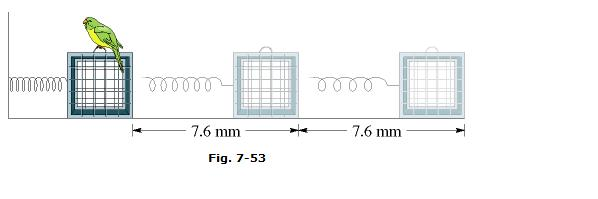# Work Done by Spring

McAfee

## Homework Statement

A spring with a spring constant of 10 N/cm has a cage attached to one end (Fig. 7-53). Taking rightward as the positive direction, (a) how much work does the spring force do on the cage when the spring is stretched from its relaxed length by 7.6 mm? (b) How much additional work is done by the spring force when the spring is stretched by an additional 7.6 mm?W= -(½)*k*(d)^2

## The Attempt at a Solution

First, I changed the units.
d=.0076m
k=1000 N/m

For

a) W = ½*1000*x0.0076² = 0.02888 J

b) W = ½*1000*(0.0152² - 0.0076²) = 0.08664 J

I know that I did the right work but I am still wrong. Does the answer have to be negative? But the problem states that the right is positive. I can't find my mistake.

McAfee
any help?

Homework Helper

## Homework Statement

A spring with a spring constant of 10 N/cm has a cage attached to one end (Fig. 7-53). Taking rightward as the positive direction, (a) how much work does the spring force do on the cage when the spring is stretched from its relaxed length by 7.6 mm? (b) How much additional work is done by the spring force when the spring is stretched by an additional 7.6 mm?W= -(½)*k*(d)^2

## The Attempt at a Solution

First, I changed the units.
d=.0076m
k=1000 N/m

For

a) W = ½*1000*x0.0076² = 0.02888 J

b) W = ½*1000*(0.0152² - 0.0076²) = 0.08664 J

I know that I did the right work but I am still wrong. Does the answer have to be negative? But the problem states that the right is positive. I can't find my mistake.

## The Attempt at a Solution

While stretching the spring to the right, you have done a positive amount of work. All that time the spring has been pulling to the left, so the spring force has been doing negative work.

McAfee
While stretching the spring to the right, you have done a positive amount of work. All that time the spring has been pulling to the left, so the spring force has been doing negative work.

Thanks a lot for helping making this clear for me.